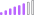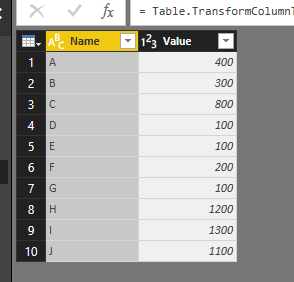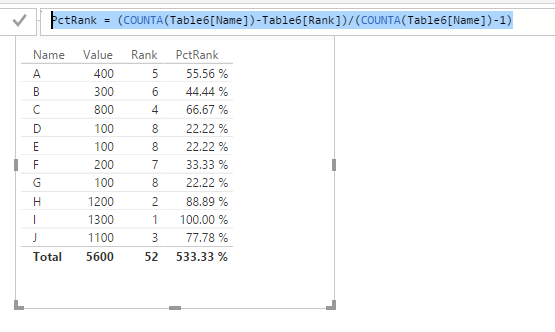cancel
Showing results for
Did you mean:Post Patron

## PERCENTRANK (Inclusive)

Excel has a PERCENTRANK function (which is different from PERCENTILE functions). I would like to find the same thing for DAX. Can anyone help me figure this out? Thank you.

1 ACCEPTED SOLUTIONSuper User

Matt - I believe the PERCENTILE functions in Excel and Power BI are similar in that they return the value sitting at a given percentile.

e.g. Excel's PERCENTILE.INC( <array>, k ) is equivalent to Power BI's PERCENTILE.INC( <column>, k ).

If k = 0.5 then they would return the 50th percentile.

PERCENTRANK in Excel is the inverse of PERCENTILE in that, for a given value (that may not appear in the array) it returns the rank expressed as a percentage.

I had a go at replicating PERCENTRANK.INC in DAX.

Sample PBIX here.

The DAX code looks like this (excessive use of variables)
Could be some bugs but works for sample data.

```PercentRank.INC =
VAR PercentRankArgument = [PercentRank Argument]
RETURN
IF (
// Only evaluate PercentRank for values between min/max of Number[Number] inclusive
AND (
PercentRankArgument >= MIN ( Number[Number] ),
PercentRankArgument <= MAX ( Number[Number] )
),
// Filter Number to values less than the PercentRankArgument
VAR NumberLessThanArgument =
FILTER ( Number, Number[Number] < PercentRankArgument )
VAR NumberGreaterThanOrEqualArgument =
FILTER ( Number, Number[Number] >= PercentRankArgument )
// RankLower = the count of Numbers less than PercentRankArgument, and is used later for interpolation of ranks
VAR RankLower =
COUNTROWS ( NumberLessThanArgument )
// NumberLower = the largest Number < PercentRankArgument, used for interpolation
VAR NumberLower =
MAXX ( NumberLessThanArgument, Number[Number] )
// NumberUpper = the smallest Number >= PercentRankArgument, used for interpolation
VAR NumberUpper =
MINX ( NumberGreaterThanOrEqualArgument, Number[Number] )
// PercentRankArgumentRank =  the rank of PercentRankArgument over the Number table, which is just RankLower + 1.
// This is the same rank as NumberUpper in the Number table itself.
VAR PercentRankArgumentRank = RankLower + 1
// InterpolationFraction = fraction that PercentRankArgument is from NumberLower to NumberUpper
VAR InterpolationFraction =
DIVIDE ( PercentRankArgument - NumberLower, NumberUpper - NumberLower )
// Calculate the interpolated rank
VAR RankInterpolated = RankLower
+ InterpolationFraction
* ( PercentRankArgumentRank - RankLower )
// Get the count of Numbers
VAR NumberCount =
COUNT ( Number[Number] )
// Final PercentRank is (RankInterpolated - 1)/(NumberCount - 1)
VAR PercentRankOutput =
DIVIDE ( RankInterpolated - 1, NumberCount - 1 )
RETURN
PercentRankOutput
```

Owen Auger
Blog
6 REPLIES 6Microsoft

@robarivas

I think you just want to calculate the PERCENTERANK for current row value. Based on the logic for PERCENTRANK, we can add a RANK column in table and achieve same logic based on this column. Please see my sample below:Create a rank column.

`Rank = RANKX(Table6,Table6[Value])`

Then create the PctRank based on the rank column.

`PctRank = (COUNTA(Table6[Name])-Table6[Rank])/(COUNTA(Table6[Name])-1)`Regards,Helper I

@v-sihou-msft Too bad PowerBI does not have the equivalent of the PercentRank function of Excel! I like your simple approach of using Rank and a computation of the Percentile. However, are you sure about your code for RankX?? It is not working when I try to duplicate your table. I get an error with your formula. Then when I try to modify it with a calculate and sum function, I only get '1' for each row!

Rank = RANKX('Table',CALCULATE(SUM('Table'[Value])))New Member

I want to see a grouping by percentile

0-20%

20-40%

40-60%

60-80%

80-100%

based on PctRank so I can see which 'Name' fall within each percentile group.New Member
I actually answered my own question with this formula 🙂 Switch = SWITCH(TRUE(), AND(Table6[PctRank]>0,Table6[PctRank]<=0.20),"0-20%", AND(Table6[PctRank]>0.20,Table6[PctRank]<=0.40),"20-40%", AND(Table6[PctRank]>0.40,Table6[PctRank]<=0.60),"40-60%", AND(Table6[PctRank]>0.60,Table6[PctRank]<=0.80),"60-80%", (Table6[PctRank]>0.80),"80-100%")As I understand, percentrank in Excel takes a score and returns its percentile. This seems to be exactly what percentile in DAX does.  Percentile in Excel does something different however.

https://msdn.microsoft.com/en-us/library/dn802531.aspx

* Matt is a Microsoft MVP (Power BI) and author of the Power BI Book Supercharge Power BI.Super User

Matt - I believe the PERCENTILE functions in Excel and Power BI are similar in that they return the value sitting at a given percentile.

e.g. Excel's PERCENTILE.INC( <array>, k ) is equivalent to Power BI's PERCENTILE.INC( <column>, k ).

If k = 0.5 then they would return the 50th percentile.

PERCENTRANK in Excel is the inverse of PERCENTILE in that, for a given value (that may not appear in the array) it returns the rank expressed as a percentage.

I had a go at replicating PERCENTRANK.INC in DAX.

Sample PBIX here.

The DAX code looks like this (excessive use of variables)
Could be some bugs but works for sample data.

```PercentRank.INC =
VAR PercentRankArgument = [PercentRank Argument]
RETURN
IF (
// Only evaluate PercentRank for values between min/max of Number[Number] inclusive
AND (
PercentRankArgument >= MIN ( Number[Number] ),
PercentRankArgument <= MAX ( Number[Number] )
),
// Filter Number to values less than the PercentRankArgument
VAR NumberLessThanArgument =
FILTER ( Number, Number[Number] < PercentRankArgument )
VAR NumberGreaterThanOrEqualArgument =
FILTER ( Number, Number[Number] >= PercentRankArgument )
// RankLower = the count of Numbers less than PercentRankArgument, and is used later for interpolation of ranks
VAR RankLower =
COUNTROWS ( NumberLessThanArgument )
// NumberLower = the largest Number < PercentRankArgument, used for interpolation
VAR NumberLower =
MAXX ( NumberLessThanArgument, Number[Number] )
// NumberUpper = the smallest Number >= PercentRankArgument, used for interpolation
VAR NumberUpper =
MINX ( NumberGreaterThanOrEqualArgument, Number[Number] )
// PercentRankArgumentRank =  the rank of PercentRankArgument over the Number table, which is just RankLower + 1.
// This is the same rank as NumberUpper in the Number table itself.
VAR PercentRankArgumentRank = RankLower + 1
// InterpolationFraction = fraction that PercentRankArgument is from NumberLower to NumberUpper
VAR InterpolationFraction =
DIVIDE ( PercentRankArgument - NumberLower, NumberUpper - NumberLower )
// Calculate the interpolated rank
VAR RankInterpolated = RankLower
+ InterpolationFraction
* ( PercentRankArgumentRank - RankLower )
// Get the count of Numbers
VAR NumberCount =
COUNT ( Number[Number] )
// Final PercentRank is (RankInterpolated - 1)/(NumberCount - 1)
VAR PercentRankOutput =
DIVIDE ( RankInterpolated - 1, NumberCount - 1 )
RETURN
PercentRankOutput
```

Owen Auger